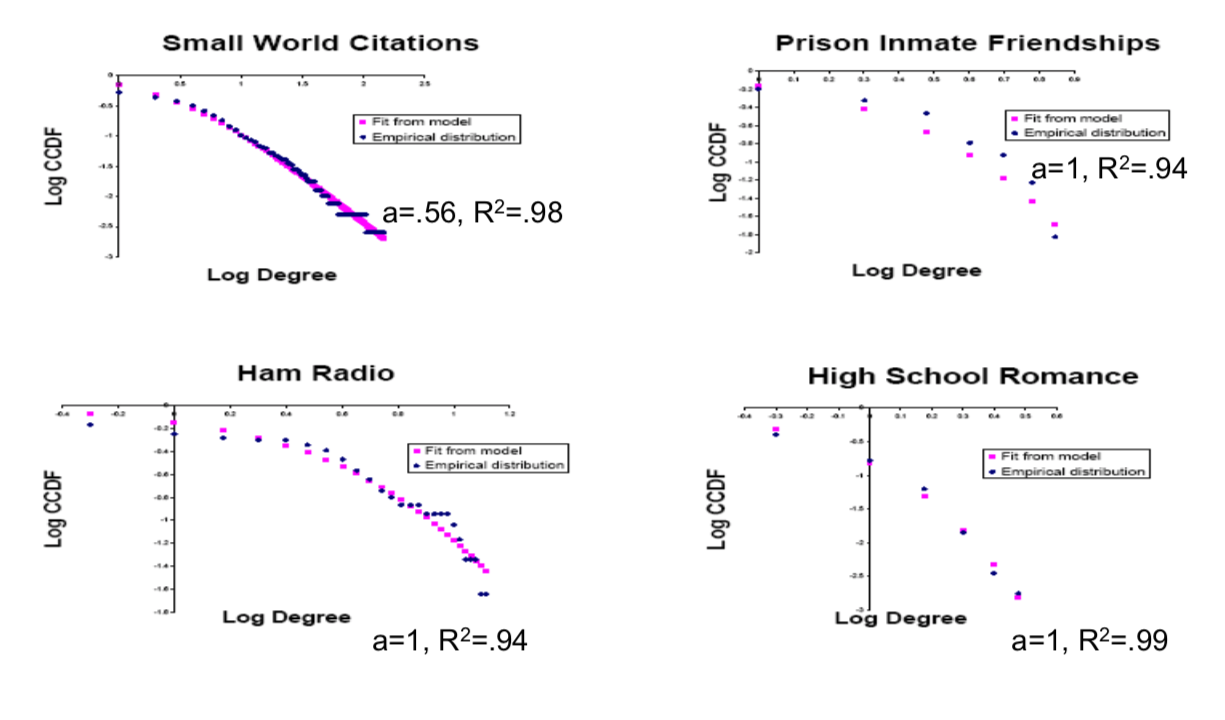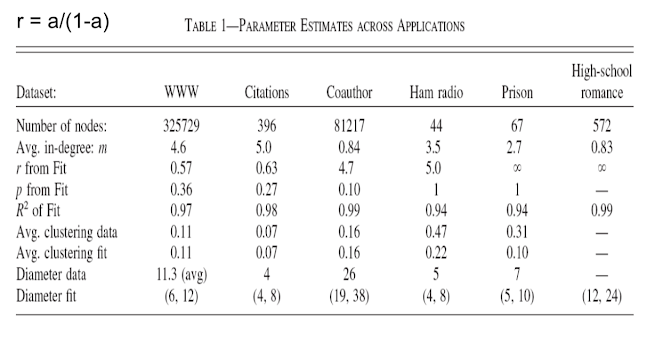# 3.5 Fitting Hybrid Models¶

## Fitting to data:¶

• $F(d) = 1 - ((m + amx) / (d + amx))^x$ where $x = 2 / (1-a)$
• $log(1 - F(d)) = c - xlog(d + amx)$
• estimate m directly
• select a to minimize distance between actual distribution and model's distribution
• minimize $\sum(F(d) - F_a(d))^2$# Notes on Fits:¶

• Ham, Prison, Romance are even more curved than with a=1 : random without growth fits even better (Poisson)

• Citations: too many with degree 0, here start with some degree# Preferential Attachment?¶

• Fit of Barabasi and Albert has a=.36

• More than 1/3 at random

• Eyeballing Log‐Log plots can be misleading!!

• Fat Tails – Yes, Actual Power law ‐ No

## Fitting the friends of friends model¶

• Fit to estimate ratio of random to network basedlinks: $r = m_r / m_n$
• $r = a/(1-a)$ from before
• r range from 0 to infinity, while a ranges from 0 to 1

## Friends of Friends/Hybrid Models:¶

• Variety of degree distributions
• Tie degree distributions to way in which links formed:
• fat tail from network meeting process
• more likely to meet well-connected nodes
• Clustering from network meeting process
• connecting to friends of friends
• this part, the clustering, comes out of the friends of friends part.
• Diameter naturally as small as E-R network
• This just comes out of the fact that we've got random network.
• Assortativity in degree based on age.
• This is something which is going to come out of any of these growing systems. So this comes out of the growth.

And it ties the degree distribution to the way that links were formed in terms of having some meeting friends of friends. But to emphasize some other things things that were missing from the original models including a straight preferential attachment model is you still don't get clustering you're just forming links at random and the chance that two of my friends were already connected to each other begins to vanish as time becomes large. When you actually do things by this Friends of Friends model then what you end up doing is connecting to people in ways that form triangles. So let me just sort of give you an illustration of that so you understand it's an important idea. And so the idea, recall here when we've got these nodes, so we've got a new node and let's say suppose we have some existing nodes and let's suppose that those existing nodes were already connected to each other. Right. So this network and the way in which the node form some attachments was first found one uniformly at random it found this node and then it attached to one of its friends. So the fact that the attachment was via this search process of finding a friend of a friend means that we've actually formed a triple here. And so we ended up with two of this nodes friends being friends with each other partly because the way it found people was through finding friends of friends. So we get natural clustering directly through this process and that's different than what happens in the peer preferential attachment model or the uniformly at random model. So the fact that you're doing things through network search gives clustering directly. So the clustering is going to depend on this a parameter but we'll find clustering in the data.

Second thing is diameter is naturally going to be small partly because you've already got either you know you've got random network formation so you've either got something that looks uniformly at random or preferential which even has a lower diameter than Erdos-Renyi kinds of networks.

Another thing is $assortativity_$. What does assortativity mean? Let's think of the older nodes. The older nodes are more likely to be connected to each other because when they were born and young the formation process was only between the ones that were existing at that time period and so older nodes are more likely to be connected to other older nodes. And so what we and up with is a correlation in the age of nodes in connections which also means that they are the ones that have a higher degree are going to tend to be connected to other ones that have high degree and ones that have lower degree are going to have more connections to ones with lower degree because they're forming ones later on their forming their links later on and so will tend to have more links to younger nodes too. And so what we'll end up with is a degree distribution that exhibits some correlation in degrees. More of a high degree node's friends will be high degree and more low degree's nodes will be low degree. So we get a series of other things coming out directly.

So we've got the growth that's giving us assortativity, the randomness gives us small world diameter, and the clustering part, the other part of small worlds is coming from the friends of friends of the process. So we're going to get three extra things.So looking at these different datasets and then you can look at the number of nodes the average in-degree, m. r, let's just instead of keeping track a, let's let r be the relatives fraction of uniformly at random compared to preferential attachment or finding friends of friends part. So as r becomes huge then it's really a random network as r goes to zero then it's pretty much pure preferential attachment. And so you get these different r's. You know some of them going off to infinity looking like random networks some pretty much lower and looking like more preferential attachment. Then you can you know look at the fit of these things. You can also look at the clustering that comes out and the clustering actually from this model fits remarkably well some of them, the fit isn't doing as well it's not generating quite as much clustering as overall but for some of them it hits it remarkably closely. The diameters, the actual diameters in the data. The diameter is from fits what you have to do is simulate the model and then you get some range of things. The ranges of the diameters are usually fairly well on. So this model also fits on a series of other dimensions besides just matching the degree distribution which is also interesting. Okay, so that takes us through a look at some of these growing random network models and what they've done is they've enriched the system to allow us to capture a series of other attributes of the actual observed networks. We can produce a variety of different degree distributions. We can take these things to data and actually estimate different parameters, see how well the models are matching some of the data. And it gives us an idea of how the actual attributes that we see in the real world might have come from some formation process. So we see a richer idea here now of how exactly we can generate these particular attributes of social networks and why they might be arising. So our next subject now, we'll be looking at some other classes of models and understanding statistical estimation of those models.